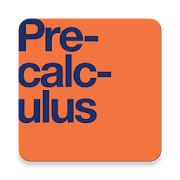Precalculus Textbook, MCQ, Test BankEveryone
6
Precalculus is adaptable and designed to fit the needs of a variety of precalculus courses. It is a comprehensive text that covers more ground than a typical one- or two-semester college-level precalculus course. The content is organized by clearly-defined learning objectives, and includes worked examples that demonstrate problem-solving approaches in an accessible way.

* Complete Textbook by OpenStax
* Multiple Choices Questions (MCQ)
* Essay Questions Flash Cards
* Key-Terms Flash Cards

https://www.quizover.com

1. Functions
Introduction to Functions
1.1. Functions and Function Notation
1.2. Domain and Range
1.3. Rates of Change and Behavior of Graphs
1.4. Composition of Functions
1.5. Transformation of Functions
1.6. Absolute Value Functions
1.7. Inverse Functions
2. Linear Functions
Introduction to Linear Functions
2.1. Linear Functions
2.2. Graphs of Linear Functions
2.3. Modeling with Linear Functions
2.4. Fitting Linear Models to Data
3. Polynomial and Rational Functions
Introduction to Polynomial and Rational Functions
3.1. Complex Numbers
3.3. Power Functions and Polynomial Functions
3.4. Graphs of Polynomial Functions
3.5. Dividing Polynomials
3.6. Zeros of Polynomial Functions
3.7. Rational Functions
3.9. Modeling Using Variation
4. Exponential and Logarithmic Functions
Introduction to Exponential and Logarithmic Functions
4.1. Exponential Functions
4.2. Graphs of Exponential Functions
4.3. Logarithmic Functions
4.4. Graphs of Logarithmic Functions
4.5. Logarithmic Properties
4.6. Exponential and Logarithmic Equations
4.7. Exponential and Logarithmic Models
4.8. Fitting Exponential Models to Data
5. Trigonometric Functions
Introduction to Trigonometric Functions
5.1. Angles
5.2. Unit Circle: Sine and Cosine Functions
5.3. The Other Trigonometric Functions
5.4. Right Triangle Trigonometry
6. Periodic Functions
Introduction to Periodic Functions
6.1. Graphs of the Sine and Cosine Functions
6.2. Graphs of the Other Trigonometric Functions
6.3. Inverse Trigonometric Functions
7. Trigonometric Identities and Equations
Introduction to Trigonometric Identities and Equations
7.1. Solving Trigonometric Equations with Identities
7.2. Sum and Difference Identities
7.3. Double-Angle, Half-Angle, and Reduction Formulas
7.4. Sum-to-Product and Product-to-Sum Formulas
7.5. Solving Trigonometric Equations
7.6. Modeling with Trigonometric Equations
8. Further Applications of Trigonometry
Introduction to Further Applications of Trigonometry
8.1. Non-right Triangles: Law of Sines
8.2. Non-right Triangles: Law of Cosines
8.3. Polar Coordinates
8.4. Polar Coordinates: Graphs
8.5. Polar Form of Complex Numbers
8.6. Parametric Equations
8.7. Parametric Equations: Graphs
8.8. Vectors
9. Systems of Equations and Inequalities
Introduction to Systems of Equations and Inequalities
9.1. Systems of Linear Equations: Two Variables
9.2. Systems of Linear Equations: Three Variables
9.3. Systems of Nonlinear Equations and Inequalities: Two Variables
9.4. Partial Fractions
9.5. Matrices and Matrix Operations
9.6. Solving Systems with Gaussian Elimination
9.7. Solving Systems with Inverses
9.8. Solving Systems with Cramer's Rule
10. Analytic Geometry
Introduction to Analytic Geometry
10.1. The Ellipse
10.2. The Hyperbola
10.3. The Parabola
10.4. Rotation of Axes
10.5. Conic Sections in Polar Coordinates
11. Sequences, Probability and Counting Theory
11.1. Sequences and Their Notations
11.2. Arithmetic Sequences
11.3. Geometric Sequences
11.4. Series and Their Notations
11.5. Counting Principles
11.6. Binomial Theorem
11.7. Probability
12. Introduction to Calculus
12.1. Finding Limits: Numerical and Graphical Approaches
12.2. Finding Limits: Properties of Limits
12.3. Continuity
12.4. Derivatives
Collapse

Reviews

Review Policy
4.7
6 total
5
4
3
2
1

Updated
March 20, 2018
Size
6.4M
Installs
5,000+
Current Version
2.1.1
Requires Android
4.4 and up
Content Rating
Everyone
Interactive Elements
Users Interact
Permissions
Offered By
QuizOver.com